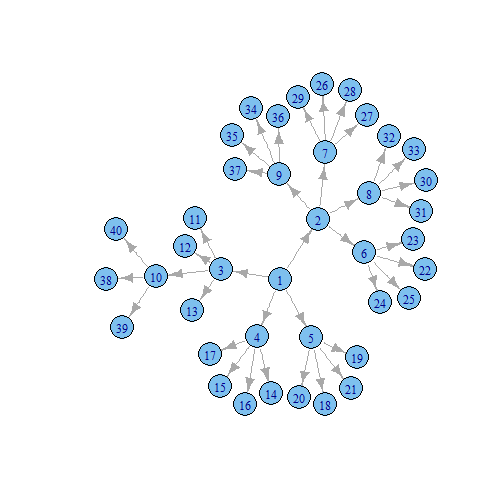﻿ Sankey from Scratch# Sankey from Scratch using `rCharts`, `d3.js`, and `igraph`

## Introduction

This example will walk through the steps of using the `R` package `igraph` to create a tree network for a sankey diagram. This is a great exercise to learn some basics of `igraph`, explore the construction of a sankey, and determine the conditions for a network to be drawn properly as a sankey. After all of this, we will plot our network with the `rCharts` implementation of the d3.js sankey plugin.

## Build a Network

Let's first start by loading the `igraph` and `rCharts` packages. Then we will use `graph.tree` to build a tree network with 40 vertices with 4 children.

``````require(igraph)
require(rCharts)

g <- graph.tree(40, children = 4)
``````

For fun, we will assign a weight of 1 `E(g)\$weight = 1` for each edge and then draw a Sankey diagram using `rCharts`. Going forward I will try to explain the `R` code through comments in the code block.

## Plot Our Sankey to Find a Problem

``````E(g)\$weight = 1

edgelist <- get.data.frame(g) #this will give us a data frame with from,to,weight
colnames(edgelist) <- c("source","target","value")
#make character rather than numeric for proper functioning
edgelist\$source <- as.character(edgelist\$source)
edgelist\$target <- as.character(edgelist\$target)

sankeyPlot <- rCharts\$new()
sankeyPlot\$setLib('libraries/widgets/d3_sankey')
sankeyPlot\$setTemplate(script = "libraries/widgets/d3_sankey/layouts/chart.html")

sankeyPlot\$set(
data = edgelist,
nodeWidth = 15,
layout = 32,
width = 960,
height = 500
)

sankeyPlot\$print(chartId = 'sankey1')
``````

Interact with the sankey plot a little, and try to find the problem in our hastily constructed network. Hovering over the vertex 4 will reveal our issue. The edge from 1 to 4 is not as big as the sum of the edges going out from 4. For this to make sense, unless 4 is magically creating something, the sum of the inflow should equal the sum of the outflow. Since 4 has four children each with weight of 1 (outflow = 1 + 1 + 1 + 1 = 4), we would expect the inflow to also be 4. It is only 1 though since we made all our edges' weights = 1 `E(g)\$weight = 1`. How then would we build our network with edge weights so that for each vertex, the sum of in equals the sum of out.

## Fix Our Problem For a Beautiful Sankey

I am a network novice, so while the code below works, I am sure there are better ways of accomplishing the desired result. I heavily commented the code, but I will quickly describe the steps. The code starts at the lowest level of the heirarchy, or those vertexes where there is nothing going out (out degree = 0). In our network, these are 11 through 40. With `igraph` we can identify these by `V(g2)[degree(g2,mode="out")==0]`. For these we will assign a weight. Then we will loop through all of the edges summing all of the weights of the out until we have reached the top of the heirarchy.

``````g2 <- graph.tree(40, children=4)
#to construct a sankey the weight of each vertex should be the sum
#of its outgoing edges
#I believe the first step in creating a network that satisfies this condition
#is define a vertex weight for all vertexes with out degree = 0
#but first let's define 0 for all
V(g2)\$weight = 0
#now for all vertexes with out degree = 0
V(g2)[degree(g2,mode="out")==0]\$weight <- runif(n=length(V(g2)[degree(g2,mode="out")==0]),min=0,max=100)
#the lowest level of the heirarchy is defined with a random weight
#with the lowest level defined we should now be able to sum the vertex weights
#to define the edge weight
#E(g2)\$weight = 0.1 #define all weights small to visually see as we build sankey
E(g2)[to(V(g2)\$weight>0)]\$weight <- V(g2)[V(g2)\$weight>0]\$weight
#and to find the neighbors to the 0 out degree vertex
#we could do V(g2)[nei(degree(g2,mode="out")==0)]
#we have everything we need to build the rest by summing
#these edge weights if there are edges still undefined
#so set up a loop to run until all edges have a defined weight
while(max(is.na(E(g2)\$weight))) {
#get.data.frame gives us from, to, and weight
#we will get this to make an easier reference later
df <- get.data.frame(g2)
#now go through each edge and find the sum of all its subedges
#we need to check to make sure out degree of its "to" vertex is not 0
#or we will get 0 since there are no edges for vertex with out degree 0
for (i in 1:nrow(df)) {
x = df[i,]
#sum only those with out degree > 0 or sum will be 0
if(max(df\$from==x\$to)) {
E(g2)[from(x\$from) & to(x\$to)]\$weight = sum(E(g2)[from(x\$to)]\$weight)
}
}
}

edgelistWeight <- get.data.frame(g2)
colnames(edgelistWeight) <- c("source","target","value")
edgelistWeight\$source <- as.character(edgelistWeight\$source)
edgelistWeight\$target <- as.character(edgelistWeight\$target)

sankeyPlot2 <- rCharts\$new()
sankeyPlot2\$setLib('libraries/widgets/d3_sankey')
sankeyPlot2\$setTemplate(script = 'libraries/widgets/d3_sankey/layouts/chart.html')

sankeyPlot2\$set(
data = edgelistWeight,
nodeWidth = 15,
layout = 32,
width = 960,
height = 500
)

sankeyPlot2
``````

## Another Look at Our Network

There are very good examples illustrating the use of `igraph` to plot a network. This is not one of these examples. For fun though, let's plot the network with igraph using just the defaults to compare it to our Sankey output from above.

``````plot(g2)
``````## Lots More Sankey

Believe it or not, there is an entire site devoted to sankey diagrams. For all the sankey you can handle, check out http://sankey-diagrams.com. Here are a couple more sankeys generated from `rCharts`: http://rcharts.io/viewer/?6001601#.UeWfuY3VCSo, http://rcharts.io/viewer/?6003605, http://rcharts.io/viewer/?6003575.